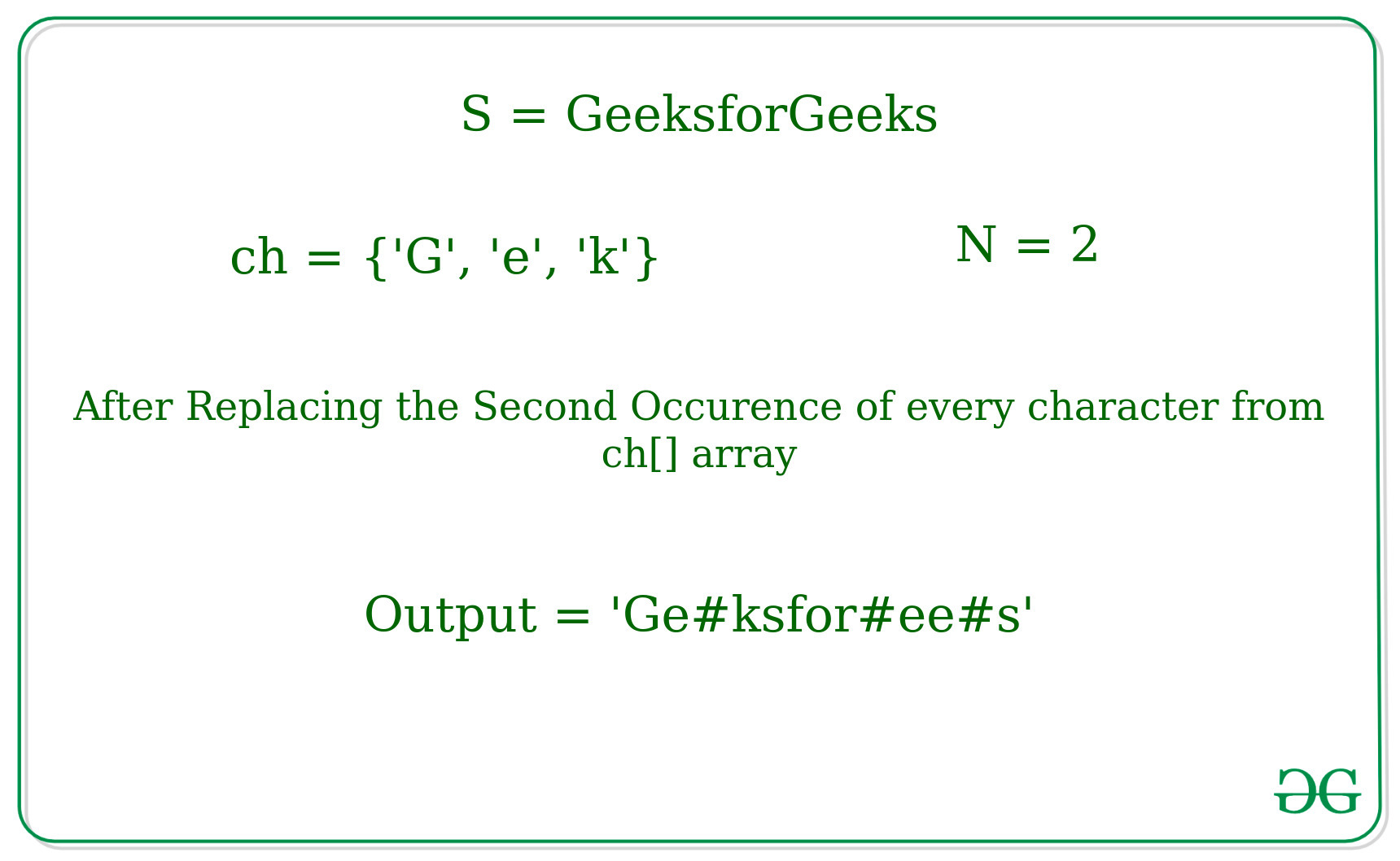# Python | Replacing Nth occurrence of multiple characters in a String with the given character

Given a String S, a character array ch[], a number N and a replacing character, the task is to replace every Nth occurence of each character of the character array ch[] in the string with the given replacing character.

Input: S = “GeeksforGeeks”, ch[] = {‘G’, ‘e’, ‘k’}, N = 2, replacing_character = ‘#’
Output: Ge#ksfor#ee#s
Explanation:
In the given string S, the second occurence of the ‘G’, ‘e’, ‘K’ is replaced with ‘#’Input: S = abcdeahu, ch[] = {‘a’, ‘d’, ‘u’}, N = 1, replacing_character = ‘#’
Output: #bc#eah#
Explanation:
In the given string S, the first occurence of the ‘a’, ‘d’, ‘u’ is replaced with ‘#’

Method 1: Naive approach
In this approach, the general idea is to store every Nth Occurrence index of every character in another array and replacing them with the given another character.

Below is the implementation of the above approach.

## Python

 `# Python implementation to replace nth ` `# occurrence of the every character ` `# in a string ` ` `  `# Function to replace the Nth occurence ` `# of the character of string ` `def` `replacer(initial_string, ch,  ` `         ``replacing_character, occurrence): ` `     `  `    ``# breaking a string into it's ` `    ``# every single character in list ` `    ``lst1 ``=` `list``(initial_string) ` `    ``lst2 ``=` `list``(ch) ` `     `  `    ``# List to store the indexes in which ` `    ``# it is replaced with the  ` `    ``# replacing_character ` `    ``lst3 ``=` `[] ` `     `  `    ``# Loop to find the Nth occurence of ` `    ``# given characters in the string ` `    ``for` `i ``in` `lst2: ` `        ``if``(lst1.count(i)>``=` `occurrence): ` `            ``count ``=` `0` `            ``for` `j ``in` `range``(``0``, ``len``(initial_string)): ` `                ``if``(i ``=``=` `lst1[j]): ` `                    ``count``+``=` `1` `                    ``if``(count ``=``=` `occurrence): ` `                        ``lst3.append(j) ` `     `  `    ``for` `i ``in` `lst3: ` `        ``# Replacing that particular index ` `        ``# with the requested character  ` `        ``lst1[i] ``=` `replacing_character ` `     `  `         `  `    ``print``(''.join(lst1)) ` `     `  `# Driver Code: ` `if` `__name__ ``=``=` `'__main__'``: ` `    ``initial_string ``=` `'GeeksforGeeks'` `    ``ch ``=` `[``'G'``, ``'e'``, ``'k'``] ` `    ``occurence ``=` `2` `    ``replacing_character ``=` `'#'` `    ``replacer(initial_string, ch, ` `    ``replacing_character, occurence) `

Output:

```Ge#ksfor#ee#s
```

Method 2: Using find() method
In this approach, the idea is to use the find() function to find the Nth occurence of the charcter in the given string S and replace it with another giver charcter.

Below is the implementation of the above approach.

## Python

 `# Python implementation to replace nth ` `# occurrence of the every character ` `# in a string using find() function ` ` `  `# Function to replace the Nth occurence ` `# of the character of string ` `def` `replacer(initial_string, ch,  ` `         ``replacing_character, occurrence): ` `     `  `    ``# breaking a string into  ` `    ``# it's every single character ` `    ``lst1 ``=` `list``(initial_string) ` `    ``lst2 ``=` `list``(ch) ` ` `  `    ``# Loop to find the occurrence ` `    ``# of the character in the string ` `    ``# and replace it with the given  ` `    ``# replacing_character ` `    ``for` `i ``in` `lst2: ` `        ``sub_string ``=` `i ` `        ``val ``=` `-``1` `        ``for` `i ``in` `range``(``0``, occurrence): ` `            ``val ``=` `initial_string.find(sub_string, ` `                                      ``val ``+` `1``)  ` `        ``lst1[val] ``=` `replacing_character ` ` `  `    ``print``(''.join(lst1)) ` ` `  `# Driver Code: ` `if` `__name__ ``=``=` `'__main__'``: ` `    ``initial_string ``=` `'GeeksforGeeks'` `    ``ch ``=` `[``'G'``, ``'e'``, ``'k'``] ` `    ``occurence ``=` `2` `    ``replacing_character ``=` `'#'` `    ``replacer(initial_string, ch, ` `    ``replacing_character, occurence) `

Output:

```Ge#ksfor#ee#s
```

Method 3: Using startswith() method
In this approach, the idea is to use the startswith() function of the python to find the index of the charcter where the occurence of the character is equal to the given Nth occurence and then replace with the given replacing character.

Below is the implementation of the above approach.

## Python

 `# Python implementation to replace nth ` `# occurrence of the every character ` `# in a string ` ` `  `# Function to replace the Nth occurence ` `# of the character of string ` `def` `replacer(initial_string, ch,  ` `         ``replacing_character, occurrence): ` `     `  `    ``# breaking a string into  ` `    ``# it's every single character ` `    ``lst1 ``=` `list``(initial_string) ` `    ``lst2 ``=` `list``(ch) ` `     `  `    ``# Loop to find the occurrence ` `    ``# of the character in the string ` `    ``# and replace it with the given  ` `    ``# replacing_character     ` `    ``for` `j ``in` `lst2: ` `        ``sub_string ``=` `j ` `        ``checklist ``=` `[i ``for` `i ``in` `range``(``0``, ``len``(initial_string))  ` `              ``if` `initial_string[i:].startswith(sub_string)]  ` `        ``if` `len``(checklist)>``=` `occurrence: ` `            ``lst1[checklist[occurrence``-``1``]] ``=` `replacing_character ` ` `  `    ``print``(''.join(lst1)) ` ` `  `# Driver Code: ` `if` `__name__ ``=``=` `'__main__'``: ` `    ``initial_string ``=` `'GeeksforGeeks'` `    ``ch ``=` `[``'G'``, ``'e'``, ``'k'``] ` `    ``occurence ``=` `2` `    ``replacing_character ``=` `'#'` `    ``replacer(initial_string, ch, ` `    ``replacing_character, occurence) `

Output:

```Ge#ksfor#ee#s
```

Attention reader! Don’t stop learning now. Get hold of all the important DSA concepts with the DSA Self Paced Course at a student-friendly price and become industry ready.

My Personal Notes arrow_drop_upCheck out this Author's contributed articles.

If you like GeeksforGeeks and would like to contribute, you can also write an article using contribute.geeksforgeeks.org or mail your article to contribute@geeksforgeeks.org. See your article appearing on the GeeksforGeeks main page and help other Geeks.

Please Improve this article if you find anything incorrect by clicking on the "Improve Article" button below.

Article Tags :
Practice Tags :

2

Please write to us at contribute@geeksforgeeks.org to report any issue with the above content.